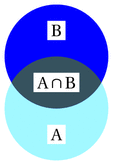Fichier f008.mp (figure 20) — Modifié le 12 Avril 2008 à 11 h 08Source

input newcourbes;
input couleur;
input geometriesyr16;
input TEX;

verbatimtex
%&latex
\documentclass{article}
\usepackage[upright]{fourier}
\usepackage{color}
\def\E{\mathrm{e}}
\let\ve\vec
\def\DR{\mathcal{D}}
\def\CR{\mathcal{C}}
\def\HR{\mathcal{H}}
\newcommand{\Mathbold}{\mbox{\boldmath$#1$\unboldmath}}
\begin{document}
etex
%%%%%%%%%%%%%%%%%%%%%%%%%%%%%%%%%%%%%%%%%%%%%%%%
%
%
%     I N T E R S E C T I ON S
%
%
%%%%%%%%%%%%%%%%%%%%%%%%%%%%%%%%%%%%%%%%%%%%%%%%%%%

beginfig(18);
path a,b,aa,ab;
a=fullcircle scaled 2cm;
b=a shifted (0,1cm);
aa=halfcircle scaled 2cm;
ab=buildcycle(aa,b);
picture pa,pb,px;
pa=thelabel(btex $A$ etex, (0,-.5cm));
pb=thelabel(btex $A\cap B$ etex, (0,0.5cm));
px=thelabel(btex $B$ etex, (0,1.5cm));

fill b withcolor white;
fill a withcolor bleu_ciel;
%fill b withcolor bleu;
%fill ab withcolor bleu_fonce;
unfill bbox pa;
draw pa;
%unfill bbox pb;
%draw pb;
%unfill bbox px;
%draw px;
endfig;

beginfig(19);
path a,b,aa,ab;
a=fullcircle scaled 2cm;
b=a shifted (0,1cm);
aa=halfcircle scaled 2cm;
ab=buildcycle(aa,b);
picture pa,pb,px;
pa=thelabel(btex $A$ etex, (0,-.5cm));
pb=thelabel(btex $A\cap B$ etex, (0,0.5cm));
px=thelabel(btex $B$ etex, (0,1.5cm));

fill a withcolor bleu_ciel;
fill b withcolor bleu;
fill ab withcolor bleu_f;
unfill bbox pa;
draw pa;
%unfill bbox pb;
%draw pb;
unfill bbox px;
draw px;
endfig;

beginfig(20);
path a,b,aa,ab;
a=fullcircle scaled 2cm;
b=a shifted (0,1cm);
aa=halfcircle scaled 2cm;
ab=buildcycle(aa,b);
picture pa,pb,px;
pa=thelabel(btex $A$ etex, (0,-.5cm));
pb=thelabel(btex $A\cap B$ etex, (0,0.5cm));
px=thelabel(btex $B$ etex, (0,1.5cm));

fill a withcolor bleu_ciel;
fill b withcolor bleu;
fill ab withcolor bleu_f;
unfill bbox pa;
draw pa;
unfill bbox pb;
draw pb;
unfill bbox px;
draw px;
endfig;

beginfig(21);
path a,b,aa,ab;
a=fullcircle scaled 2cm;
b=a shifted (0,1cm);
aa=halfcircle scaled 2cm;
ab=buildcycle(aa,b);
picture pa,pb,px,pu;
pa=thelabel(btex $A$ etex, (0,-.5cm));
pb=thelabel(btex $A\cap B$ etex, (0,0.5cm));
px=thelabel(btex $B$ etex, (0,1.5cm));
pu=thelabel(btex $A\cup B$ etex, (0,0.5cm));

fill a withcolor bleu_m;
fill b withcolor bleu_m;
fill ab withcolor bleu_m;
unfill bbox pu;
draw pu;
endfig;

end